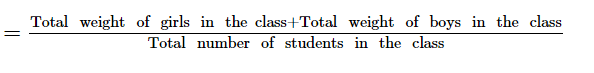# There are 50 students in a class, of which 40 are boys.Question:

There are 50 students in a class, of which 40 are boys. The average weight of the class is 44 kg an that of the girls is 40 kg. Find the average weight of the boys.

Solution:

Total students in the class = 50
Number of boys = 40
∴ Number of girls = (50 -">- 40) = 10
Average weight of students in the class = 44 kg
Average weight of girls in the class = 40 kg
Sum of the weights of girls in the class = (40 ×">××10) kg = 400 kg
Thus, we have:

Average weight of students in the class$\Rightarrow 44=\frac{400+\text { Total weight of boys in the class }}{50}$

$\Rightarrow$ Total weight of boys $+400=2200$

$\Rightarrow$ Total weight of boys $=1800 \mathrm{~kg}$

Average weight of boys $=\frac{1800}{40}=45 \mathrm{~kg}$